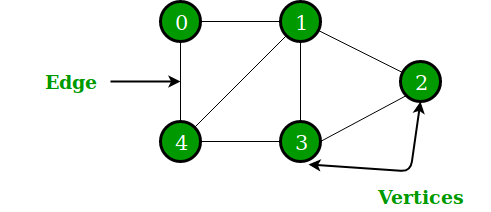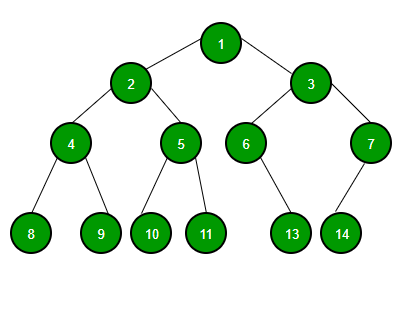Open In App
Related Articles

# Difference between graph and tree

A graph is a collection of two sets V and E where V is a finite non-empty set of vertices and E is a finite non-empty set of edges.

• Vertices are nothing but the nodes in the graph.
• Two adjacent vertices are joined by edges.
• Any graph is denoted as G = {V, E}.

For Example:G = {{V1, V2, V3, V4, V5, V6}, {E1, E2, E3, E4, E5, E6, E7}}

A graph is a collection of vertices (also known as nodes) and edges that connect these vertices. Each edge represents a relationship or connection between two vertices.

Graphs can be directed or undirected, meaning that edges have a specific direction or they do not.

A directed graph is also known as a digraph. Graphs can also have weighted edges, where each edge has a weight or cost associated with it. Graphs can be represented in various ways, such as adjacency matrix or adjacency list.

Tree :

A tree is a special type of graph that is connected and acyclic, meaning that there are no cycles in the graph.

In a tree, there is a unique path between any two vertices, and there is a single vertex called the root that is used as the starting point for traversing the tree.

Trees can be used to model hierarchical relationships, such as the structure of a file system or the organization of a company.

Trees can be binary or non-binary. In a binary tree, each node has at most two children, while in a non-binary tree, each node can have any number of children.

Binary trees can be used to solve problems such as searching and sorting, as well as to represent expressions and parse trees.

A tree is a finite set of one or more nodes such that –

1. There is a specially designated node called root.
2. The remaining nodes are partitioned into n>=0 disjoint sets T1, T2, T3, …, Tn
where T1, T2, T3, …, Tn are called the subtrees of the root.

The concept of a tree is represented by following Fig.